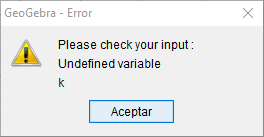# How to generate a sequence of Free Segments?

jospercomp shared this question 2 years ago

Sequence(Segment((i, 0), (i, 1)), i, 1, 5)

outputs

S_1=Segment((1, 0), (1, 1))

S_2=Segment((2, 0), (2, 1))

S_3=Segment((3, 0), (3, 1))

S_4=Segment((4, 0), (4, 1))

S_5=Segment((5, 0), (5, 1))Execute[Sequence["S_{"+(i)+"}=Segment(("+i+", 0), ("+i +", 1) )",i, 1, Length(Lcolor)]]

Execute(Sequence("ShowLabel( S_{"+i+"}, false )", i, 1, Length(Lcolor)))

Execute(Sequence("SetLineThickness(S_{"+k+"}, 5)", k, 1, Length(Lcolor)))

Execute(Sequence("SetLineStyle(S_{"+k+"}, 4)", k, 1, Length(Lcolor)))

Execute(Sequence("SetColor(S_{"+k+"}, Element(Lcolor,"+k+",1)/255, Element(Lcolor,"+ k+",2)/255, Element(Lcolor,"+ k+",3)/255)", k, 1, Length(Lcolor)))1

How are you using them? Maybe the spreadsheet is the best way but it's hard to know without the context1

Must be using Repeat and/or Execute Command?

Sequence(Segment((i, 0), (i, 1)), i, 1, 5)

This command create a list. I want to generate the separate elements creating a supercommand and label will be S_1, S_2,S_3,S_4, ... S_n1

Execute[Sequence["S_{"+(i)+"}=Segment(("+i+", 0), ("+i +", 1) )",i, 1, 10]]1

This command have error:Sequence(SetColor("S_{"+k+"}", Element(Lcolor,k,1)/255, Element(Lcolor, k,2)/255, Element(Lcolor, k,3)/255), k, 1, 10)

Sequence(SetLineThickness("S_{"+k+"}", 5), k, 1, 10)

Sequence(SetLineStyle("S_{"+k+"}", 4), k, 1, 10)

https://wiki.geogebra.org/e...1

Execute(Sequence("ShowLabel( S_{"+i+"}, false )", i, 1, 10))

Execute(Sequence("SetLineThickness(S_{"+k+"}, 5)", k, 1, 10))

Execute(Sequence("SetLineStyle(S_{"+k+"}, 4)", k, 1, 10))

Execute(Sequence("SetColor(S_{"+k+"}, Element(Lcolor,"+k+",1)/255, Element(Lcolor,"+ k+",2)/255, Element(Lcolor,"+ k+",3)/255)", k, 1, 10))2

Do you want a free list of segments (i.e., if you drag one segment around the others in the list also move), or do you want each segment to be a separate free object?

Generally you can that via Execute:

```Execute({"{"+sum(Sequence(If(i ≟ 1, "", ",") + "Segment((" + (i) + ", 0), (" + (i) + ",1))", i, 1, 5))+"}"})
Execute(Sequence("Segment(("+i+", 0), ("+i+",1))", i, 1, 5))```

And of course you can modify the string generation to generate particular object names for the second version.

For the first option I had thought that

`CopyFreeObject(Sequence(Segment((i, 0), (i, 1)), i, 1, 5))`

would work, but it surprisigly doesn't. Instead of a list of segments you get a list of lines. Seems like a bug to me, but might just be expected behaviour for some reason.1

This command have error:Sequence(SetColor("S_{"+k+"}", Element(Lcolor,k,1)/255, Element(Lcolor, k,2)/255, Element(Lcolor, k,3)/255), k, 1, 10)

Sequence(SetLineThickness("S_{"+k+"}", 5), k, 1, 10)

Sequence(SetLineStyle("S_{"+k+"}", 4), k, 1, 10)1

Lcolor={{0, 0, 159}, {0, 0, 191}, {0, 0, 223}, {0, 0, 255}, {0, 32, 255}, {0, 64, 255}, {0, 96, 255}, {0, 128, 255}, {0, 159, 255}, {0, 191, 255}, {0, 2 * 23, 255}, {0, 255, 255}, {32, 255, 223}, {64, 255, 191}, {96, 255, 159}, {128, 255, 128}, {159, 255, 96}, {191, 255, 64}, {223, 255, 32}, {255, 255, 0}, {255, 223, 0}, {255, 191, 0}, {255, 159, 0}, {255, 128, 0}, {255, 96, 0}, {255, 64, 0}, {255, 32, 0}, {255, 0, 0}, {223, 0, 0}, {191, 0, 0}}1

Execute[Sequence["S_{"+(i)+"}=Segment(("+i+", 0), ("+i +", 1) )",i, 1, Length(Lcolor)]]

Execute(Sequence("ShowLabel( S_{"+i+"}, false )", i, 1, Length(Lcolor)))

Execute(Sequence("SetLineThickness(S_{"+k+"}, 5)", k, 1, Length(Lcolor)))

Execute(Sequence("SetLineStyle(S_{"+k+"}, 4)", k, 1, Length(Lcolor)))

Execute(Sequence("SetColor(S_{"+k+"}, Element(Lcolor,"+k+",1)/255, Element(Lcolor,"+ k+",2)/255, Element(Lcolor,"+ k+",3)/255)", k, 1, Length(Lcolor)))1

Colormap reverse

Execute(Sequence("SetColor(S_{"+k+"},Element(Lcolor,Length(Lcolor)+"+(-k)+"+1,1)/255,Element(Lcolor,Length(Lcolor)+"+(-k)+"+1,2)/255,Element(Lcolor,Length(Lcolor)+"+(-k)+"+1,3)/255)", k, 1, Length(Lcolor)))1

Execute[Sequence["QR_{"+(i)+"}=Polygon({("+i+", 0),("+i+",-100),("+(i-1)+",-100),("+(i-1)+",0)"+"})",i,1,Length(Lcolor)]]

Execute(Sequence("SetLineThickness(QR_{"+k+"}, 0)", k, 1, Length(Lcolor)))

Execute(Sequence("SetColor(QR_{"+k+"},Element(Lcolor,"+k+", iCR)/255, Element(Lcolor,"+ k+", iCG)/255,Element(Lcolor, "+k+", iCB)/255)", k, 1, nr))

Execute[Sequence["SetFilling(QR_{"+(i)+"},0.8)",i,1,nr]]1

Execute[Sequence["f_{"+(i)+"}=Dilate(eq2,"+i+")",i, 1,10]]